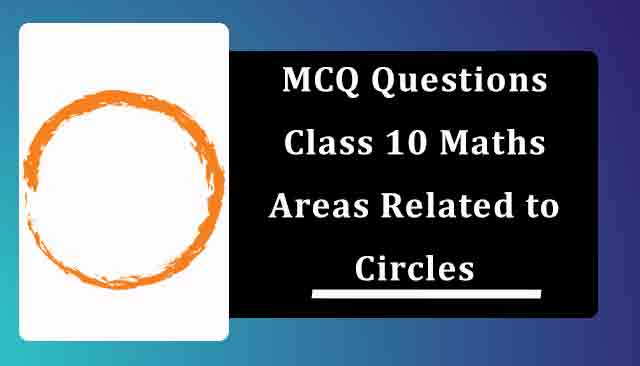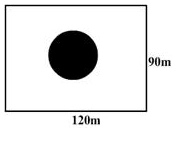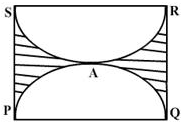# MCQ Questions for Class 10 Maths Chapter 12 Areas Related to Circles with Answers

Below you will find NCERT MCQ Questions for Class 10 Maths Chapter 12 Areas Related to Circles with Answers which is very helpful during the preparation of examinations. These MCQ Online Test are one marks questions which will give you overview of the chapter in no time. One should try to understand Class 10 MCQ Questions as it is based on latest exam pattern released by CBSE.

Also, students can check NCERT Solutions for Class 10 Maths Chapter 12 for improving their marks and have good understanding of the chapter.## Chapter 12 Areas Related to Circles MCQ Questions for Class 10 Maths with Answers

1. A park is in the form of a rectangle 120m by 90 m. At the centre of the park there is a circular lawn as shown in the figure. If the area of the park excluding the lawn is 2950 m2, then the radius of the circular lawn will be(a) 60 m
(b) 50 m
(c) 40 m
(d) 35 m
Solution (b) 50 m

2. The circumference of a circle whose diameter is 4.2 cm is
(a) 4.2 cm
(b) 22 cm
(c) 13.2cm
(d) 11 cm
Solution (c) 13.2cm

3. If PQRS is a square of side 14 cm and PAQ and SAR are semi-circles, then the area of the shaded region is(a) 80 cm2
(b) 35 cm2
(c) 42 cm2
(d) 60 cm2
Solution (c) 42 cm2

4. If the circumference of a circle increases from 2 to 4 then its area is:
(a) Doubled
(b) Tripled
(c) Four times
(d) Halved
Solution (c) Four times

5. The area of a sector of a circle bounded by an arc of length 5π cm is equal to 20π cm2, then its radius is
(a) 12 cm
(b) 16 cm
(c) 8 cm
(d) 10 cm
Solution (c) 8 cm

6. The ratio of area of two circles whose ratio of circumference is 3:1 will be:
(a) 3 : 1
(b) 1 : 3
(c) 1 : 9
(d) 9 : 1
Solution (d) 9 : 1

7. The circumference of a circle exceeds its diameter by 120 cm, then its radius is
(a) 14 cm
(b) 28 cm
(c) 46 cm
(d) 52 cm
Solution (b) 28 cm

8. What is the area of the square inscribed in a circle with a circumference of 18.84 cm?
(a) 20 cm2
(b) 18 cm2
(c) 15 cm2
(d) 16 cm2
Solution (b) 18 cm2

9. The diameter of a circle whose area is equal to the sum of the areas of the two circles of radii 24 cm and 7 cm is​
(a) 50 cm
(b) 31 cm
(c) 62 cm
(d) 25 cm
Solution (a) 50 cm

10. From a circular sheet of paper with a radius 20 cm, four circles of radius 5 cm each are cut out. What is the ratio of the uncut to the cut portion?​
(a) 3:1
(b) 4:1
(c) 1:3
(d) 4:3
Solution (a) 3:1

11. If the perimeter of a circle is equal to that of a square, then the ratio of their areas is
(a) 14 : 11
(b) 22 : 7
(c) 11 : 14
(d) 7 : 22
Solution (a) 14 : 11

12. The area of a square inscribed in a circle of diameter ‘d’ is
(a) d2/2
(b) d2/4
(c) d2
(d) d/2
Solution (a) d2/2

13. The number of rounds that a wheel of diameter 7/11 m will make in going 4 km is
(a) 1500
(b) 1700
(c) 2000
(d) 2500
Solution (c) 2000

14. The diameter of a wheel is 1 m. The number of revolutions it will make to travel a distance of 22 km will be
(a) 2,800
(b) 4,000
(c) 5,500
(d) 7,000
Solution (d) 7,000

15. The radii of two concentric circles are 19cm and 16cm. The area of the ring enclosed between them is
(a) 330 sq. cm
(b) 370 sq. cm
(c) 390 sq. cm
(d) 350 sq. cm
Solution (a) 330 sq. cm

16. The radius of the circle whose area is equal to the sum of the areas of the two circles of radii 24 cm and 7 cm is
(a) 7 cm
(b) 24 cm
(c) 25 cm
(d) 30 cm
Solution (c) 25 cm

17. A garden roller has a circumference of 4 m. The number of revolutions it makes in moving 40 metres are:
(a) 12
(b) 16
(c) 8
(d) 10
Solution (d) 10

18. The diameter of a circle whose area is equal to the sum of the areas of the two circles of radii 24 cm and 7 cm is
(a) 31 m
(b) 25 m
(c) 62 m
(d) 50 m
Solution (d) 50 m

19. If the difference between the circumference and radius of a circle is 37 m, then the circumference of the circle is given by
(a) 33 cm
(b) 55 cm
(c) 22 cm
(d) 44 cm
Solution (d) 44 cm

20. The diameter of a cycle wheel is 28 cm. The number of revolutions it makes in moving 13.2 km is​
(a) 500
(b) 8000
(c) 15000
(d) 1500
Solution (c) 15000

21. The sum of the diameters of two circles is 280 cm and the difference of their circumferences is 88 cm. Then the larger of the two radii is​
(a) 44 cm
(b) 77 cm
(c) 63 cm
(d) cannot be determined
Solution (b) 77 cm

22. The area of the circle that can be inscribed in a square of side 10cm is
(a) 20π sq.cm
(b) 25π sq.cm
(c) 25 sq.cm
(d) 10π sq.cm
Solution (b) 25π sq.cm
أحدث أقدم